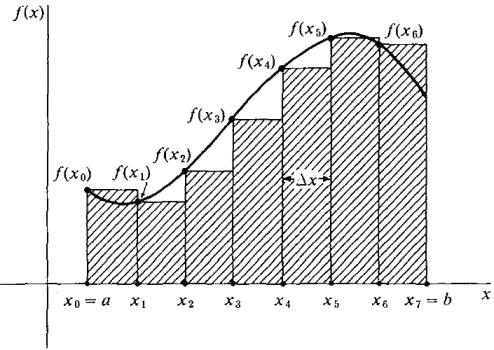# Riemann Sums

Ki-nana18

## Homework Statement

Identify an=the summation from k=1 to n of (2n)/(4k2+1) as a Riemann sum of an appropriate function on an appropriate interval and find the limit as n approaches infinity of an.

## Homework Equations

There is no interval givien so I assume its from 0 to 1.

## The Attempt at a Solution

I only got up to 2n/(4(k/n)^2+1) and I'm not sure what to do from there.

eczeno

## Homework Statement

Identify an=the summation from k=1 to n of (2n)/(4k2+1) as a Riemann sum of an appropriate function on an appropriate interval and find the limit as n approaches infinity of an.

## Homework Equations

There is no interval givien so I assume its from 0 to 1.

you cannot make this assumption. the information needed to determine the interval will be hidden in the expression given.

## The Attempt at a Solution

I only got up to 2n/(4(k/n)^2+1) and I'm not sure what to do from there.

are you sure you have the problem copied down correctly? the sum you present, with the 2n in the numerator, will not converge when you take the limit as n goes to infinity, thus it does not represent any definite integral. if it were

$$\sum_0^n{\frac{4k^2+1}{2n}}$$

it would look better, but still not converge.

A reimann sum looks like this:

$$\sum_{k=1}^n{f(x_k)}\Delta x$$

where the $x_k$ all depend on the ratio $k/n$ and $\Delta x$ depends on $1/n$. so, there should always be one more n in the denominator than there are k's in the numerator. If this is not the case, the limit will not converge.

Last edited:
Ki-nana18
I copied it down wrong. Its actually the summation from k=1 to n of (2n)/(4k^2+n^2). Can I solve this without a given interval?

eczeno
yes! but i have to go now, i will show you how later (if someone else doesn't beat me to it).

eczeno
ok, so, if i understand correctly, the sum looks like this:

$$\sum_{k=1}^n{\frac{2n}{4k^2+n^2}$$

this is a proper reimann sum, so we are good to proceed. we need to make it look like this:

$$\sum_{k=1}^n{f(x_k)\Delta x}$$

and we need to determine the interval over which we are taking the sum, that is find a and b, the limits of integration, for when we turn our sum into a definite integral.

this seems like a daunting task because we don't know the function $f$. and, well, this is a bit tricky, but we are not totally lost at sea here. we do know how $x_k$ and $\Delta x$ depend on n, a and b. do you know the formulas for $x_k$ and $\Delta x$ in the reimann sum? they depend on whether it is a right, left, or some other sum. if it is not stated, we can assume it is a right or left sum. and, we can tell which one by looking at the sum. right reimann sums take k from 1 to n, while left reimann sums take k from 0 to n-1. so, we have a right reimann sum. let me know if you need help with those formulas. we cannot proceed until you understand them pretty well.

cheers

eczenohere is an image that will help us visualize what is happening.